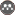Volume 16, Issue 61 (fall 2012)                   JWSS 2012, 16(61): 209-219 | Back to browse issues page

BibTeX | RIS | EndNote | Medlars | ProCite | Reference Manager | RefWorks
Send citation to:A. Vaezi, M. Abbasi. Efficiency of the SCS-CN Method in Estimating Runoff in Taham Cahi Watershed, North West of Zanjan. JWSS. 2012; 16 (61) :209-219
URL: http://jstnar.iut.ac.ir/article-1-2440-en.html
, vaezi.alireza@gmail.com
Abstract:   (15072 Views)
The Soil Conservation Service Curve Number (SCS-CN) method is widely used for predicting direct runoff from rainfall events. The ratio of initial abstraction (λ=Ia/S) to maximum potential retention (S) was assumed in its original development to be equal to 0.2 (λ=Ia/S=0.2) in SCS-CN method. Application of the initial abstraction ratio equal to 0.2 out of the area where it has been developed may lead to a non logical estimation of runoff. Thus, the study was conducted to determine the initial abstraction ratio (λ=Ia/S) by analyzing measured rainfall-runoff events. The dataset consisted of 58 rainfall-runoff events during 15 years (1987-2001) of rainfall and runoff measurements from Taham-Chay watershed, northwest of Zanjan, Iran. Based on the results, the estimated runoff value on the basis of Ia= 0.2S was 26.7 times higher than the measured value, on average. There was a very low relationship between the measured and estimated runoff values (R2=0.09) and mean model error was 0.13. The Ia/S values varied from 0.004 to 0.008 with an average of 0.006. When Ia/S value was modified to 0.08, ratio of the measured to estimate runoff value was 1.4 and the determination coefficient (R2) of the relationship between the two was 0.41. When seven rainfall events that had the low rainfall intensity values (lower than 0.14 mm/h) and two events that had the high rainfall depth (bigger than 10.47 mm) during the past five days were removed from the data analysis process, ratio of the measured to estimated runoff value decreased to 1.3 and the determination coefficient (R2) of the relationship between the two enhanced to 0.90. The mean model error for the modified Ia/S value also decreased to 0.007. It also improved model efficiency coefficient (EF) to -0.089 compared with 0.91 for traditional Ia/S value (0.2).